Courses

# Physical Chemistry MCQ

## 21 Questions MCQ Test Mock Test Series of IIT JAM Biotechnology | Physical Chemistry MCQ

Description
This mock test of Physical Chemistry MCQ for IIT JAM helps you for every IIT JAM entrance exam. This contains 21 Multiple Choice Questions for IIT JAM Physical Chemistry MCQ (mcq) to study with solutions a complete question bank. The solved questions answers in this Physical Chemistry MCQ quiz give you a good mix of easy questions and tough questions. IIT JAM students definitely take this Physical Chemistry MCQ exercise for a better result in the exam. You can find other Physical Chemistry MCQ extra questions, long questions & short questions for IIT JAM on EduRev as well by searching above.
QUESTION: 1

### The angular momentum of an electron present in 3s and 3p orbital are

Solution: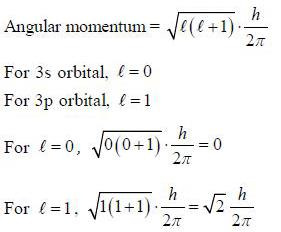QUESTION: 2

### The reduction potential at 25°C for Fe3+ Fe2+ electrode if the concentration of Fe+2 ion is five times that of Fe+3 ion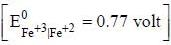Solution:

The electrode reaction is,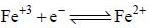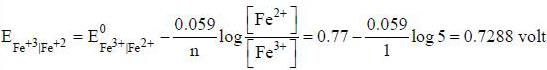QUESTION: 3

### The rate constant of a reaction is 1.5 x 107 s-1 at 50°C and 4.5 x 107 s-1 at 100°C. The Arrehenius parameter A and εa is

Solution: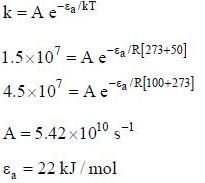QUESTION: 4

The value AH of the reaction.
CH2Cl2(g) → C(g)+2H(g) + 2Cl(g)
The average bond energies of C-H and C-Cl bonds are 416 and 328 kJ mol respectively

Solution: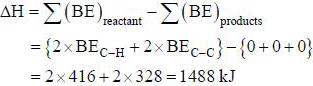QUESTION: 5

Certain mass of a gas occupy 200 mL at 127°C. If the gas is cooled to -73°C at constant pressure, its new volume is

Solution:

Charles Law.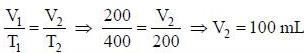QUESTION: 6

The ratio of the radius of second orbit of Li2+ ion with the radius of third orbit of He+ ion is

Solution: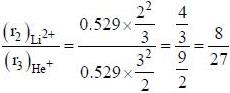QUESTION: 7

For a reaction. 2A +  B → 3Z if the rate consumption of A is 2 x 10-4 mol dm-3 s-1. The rate of formation of Z (in mol dm-3 s-1) will be

Solution:

Rate of formation of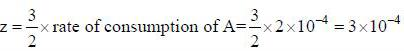QUESTION: 8

The ionic strength of a solution containing 0.008 M AlCl3 and 0.005 M KCl is

Solution: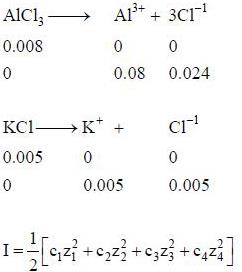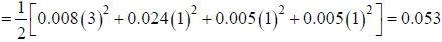QUESTION: 9

The uncertainty in measuring the position of a proton is 2 x 10-6 m. The minimum uncertainty in measuring the speed of this proton is (mass of proton is 1.672 x 10-27 kg)

Solution: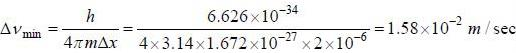QUESTION: 10

The vapour pressure of liquid decane is 10 torr at 55.7°C and 400 torr at 150.6°C. The value of ΔHv is

Solution: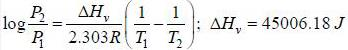*Multiple options can be correct
QUESTION: 11

The collect statement in the following is/are

(1) the correct expression for Schrodinger’s equation is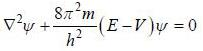(2) Bohr's quantisation condition is mvr = nh/2π
(3) The de-Broglie wavelength of an electron revolving in the second orbit of hydrogen atom is 6.649 x 10-10m
(4) Radius of nth orbit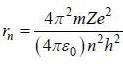Solution: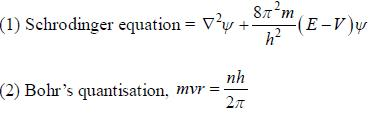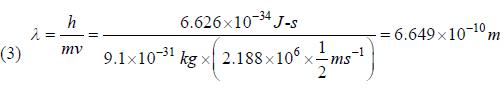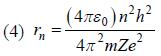*Multiple options can be correct
QUESTION: 12

Among the following the reaction that is accompanied by a increase in the entropy is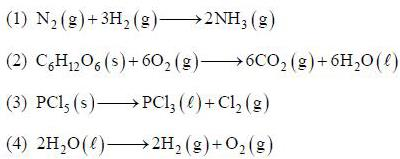The conect equation is

Solution: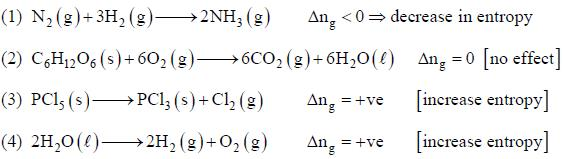*Multiple options can be correct
QUESTION: 13

For an ideal gas incorrect expresion is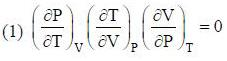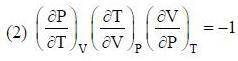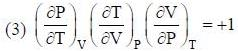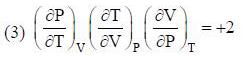Solution: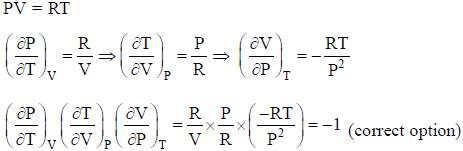*Multiple options can be correct
QUESTION: 14

Which of the following statements about catalyst is correct
(1) A catalyst increase the rate of a reaction without being destroyed
(2) A catalyst does not change the position of equilibrium
(3) A catalyst increase the rate of both forward and backward reactions
(4) A catalyst speeds up a reaction without taking pait in reaction

Solution:

A catalyst increase the rate of a reaction without being destroyed → correct
A catalyst does not change the position of equilibrium → correct
A catalyst increase the rate of both forward and backward reactions → correct
A catalyst speeds up a reaction without taking part in reaction → incorrect

*Multiple options can be correct
QUESTION: 15

The collect statement is are
(1) Debye-Huckel Onsagar equation is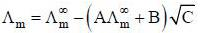(2) The unit of specific resistance is Ω-1m-1s
(3) The ionic strength of 0.15 molal KCl solution is 0.15
(4) The unit of equivalent conductance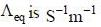Solution: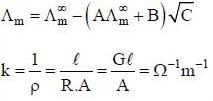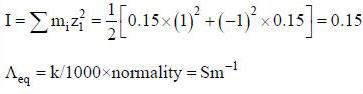*Answer can only contain numeric values
QUESTION: 16

The circumference of the second orbit of an atom or ion having single electron is 4 x 10-9 m. The de-Broglie wavelength of electron revolving in this orbit is ____10-9 m

Solution: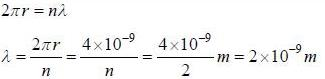*Answer can only contain numeric values
QUESTION: 17

The half life of a first order reaction is 12.6 h. The time at which the concentration of reactants becomes 0.001 M from 1.0 M is _____ h.

Solution: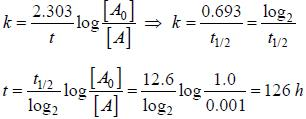*Answer can only contain numeric values
QUESTION: 18

Two moles of an ideal gas are compressed isothermally (100°C) and reversibly from a pressure of 10 atm to 25 atm. The value of free energy is ______

Solution: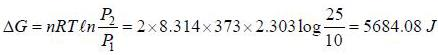*Answer can only contain numeric values
QUESTION: 19

A current of 0.5 A is passed through molten AlCl3 for 40 min the mass of aluminium deposited at the cathode is _____ g (Al = 27)

Solution: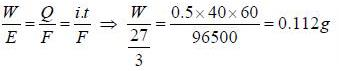*Answer can only contain numeric values
QUESTION: 20

The pH of a neutral solution at 37°C, where kω equals 2.5 x 10-14 is  ______

Solution: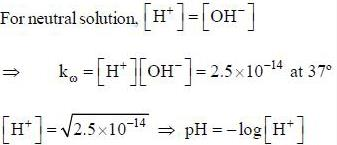*Answer can only contain numeric values
QUESTION: 21

For an enzyme substrate reaction a plot between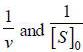yields a slope ot 40s. It the enzyme concentration is 2.5 μM. Then the catalytic efficiency of the enzyme is 10x L mol-1s-1. The value of x is _____

Solution: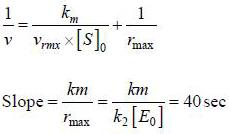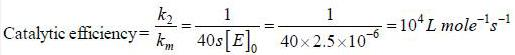Track your progress, build streaks, highlight & save important lessons and more!

### Similar Content### Related tests